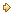Visitors Online: 77 | Friday 24th May 2019

CBSE Guess > Papers > Question Papers > Class XII > 2004 > Economics > Compartment Outside Delhi Set-I

ECONOMICS (Set I—Compartment Outside Delhi)

SECTION - A

Q. 1. Answer the following questions: 1x4
(i) State what economics is all about.
(ii) Give meaning of producer’s equilibrium.
(iii) Give one example of fixed cost.
(iv) Define market period.

Q. 2. What is ‘opportunity cost’ of a given activity? Explain with the help of an example. 3

Q. 6. A consumer spends Rs. 250 on a good when its price is 5 per unit. When the price rises to Rs. 6 per unit, he spends Rs. 240. Calculate the price elasticity by percentage method. 4

Q. 7. Explain the effect on output when only one input is increased and all other inputs are held constant. 4

Q. 8. Complete the following table: 4

 Price (Rs.) Output (Units) Total Revenue (Rs.) Marginal Revenue (Rs.) 5 6 7 8 8 7 6 5 — — — — — — — —

Q. 9. State two features common to prefect competition and monopolistic competition. Explain any one. 4
Or
State three features of monopoly. Explain any one.

Q. 10. Explain any three factors which lead to ‘decrease in demand’. 6

Q. 11. Explain the concept of ‘returns to scale’ with the help of an example. 6
Or
Explain any three determinants of supply of a good.

Q. 12. Draw Average Total Cost, Average Variable Cost and Marginal Cost curves in a single diagram. Also explain the relation between Marginal Coat and Average Total Cost with its help. 6

SECTION - B

Q. 13. Answer the following questions: 1x4
(i) Give meaning of micro economics.
(ii) Give one example of macro economics.
(iii) Define foreign exchange rate.
(iv) Give meaning of Balance of Payments.

Q. 14. Calculate Gross Value Added at Market Price from the following: 3

 (Rs. in lakhs) (i) Intermediate cost (ii) Closing stock (iii) Sales (iv) Net indirect tax (v) Subsidy (vi) Depreciation (vii) Opening stock 8 5 30 6 1 3 4

Q. 15. If marginal propensity to save is 0.1 and increase in national income is Rs. 500 crores, calculate increase in investment. 3

Q. 16. Explain briefly the meaning of ‘excess demand’ and ‘deficient demand’ in an economy. 3

Q. 18. Explain the ‘medium of exchange’ function of money. 4
Or
Explain the ‘unit of value’ function of money.

Q. 19. Explain the ‘banker to the government’ function of a central bank. 4

Q. 20. Explain the meaning of revenue expenditure and capital expenditure in a government budget with appropriate examples. 4

Q. 21. Explain any two objectives of a government budget. 4

Q. 22. Distinguish between a factor payment and a transfer payment. Giving reasons, state whether the following are included in national income or not. 6
(i) Brokerage payment on sale of shares.
(ii) Interest payment on loan taken by an individual to buy a motor cycle.
(iii) Festival gift by an employer to his employees.

Q. 23. Calculate (a) Net National Product at Market Price, (b) Gross National Disposable Income: 4, 2

 (Rs. in crores) (i) Private final consumption expenditure (ii) Net indirect tax (iii) Change in stocks (iv) Net current transfers from abroad (v) Government final consumption expenditure (vi) Consumption of fixed capital (depreciation) (vii) Net domestic capital formation (viii) Net factor income from abroad (ix) Net imports 200 20 (-) 5 (-) 10 50 15 30 5 10

Q. 24. Explain two fiscal policy measures for increasing aggregate demand in an economy. 6
Or
Explain two monetary policy measures for increasing aggregate demand in an economy.

 Economics 2004 Question Papers Class XII Delhi Outside Delhi Compartment Delhi Compartment Outside DelhiSet ISet ISet ISet ISet IISet IISet IISet IISet IIISet III

CBSE 2004 Question Papers Class XII